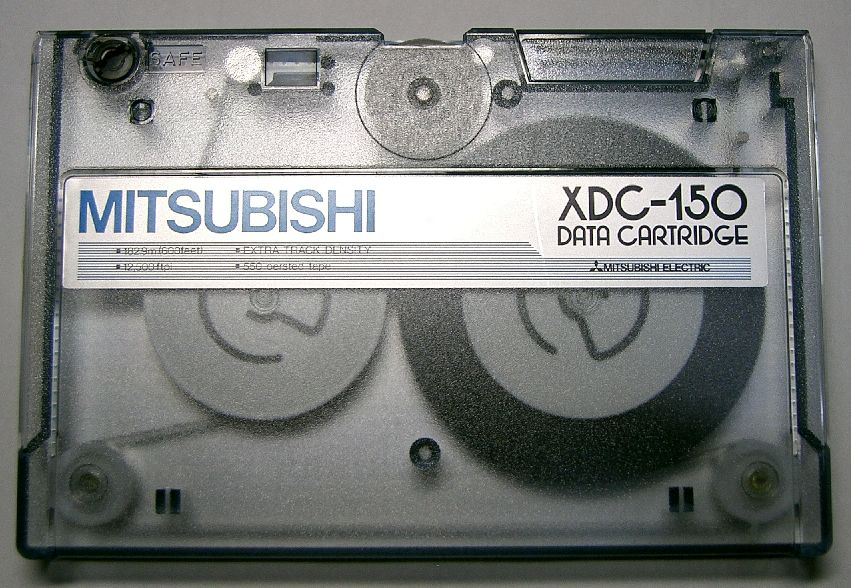# Social Network Analysis in R

Laboratory One
Benjamin Lind

Social Network Analysis: Internet Research
St. Petersburg
18 August, 2013 (11:45-13:15)Implements the S programming language
Open source
Object oriented programming language
Base & user-contributed packages
Meets practically all statistical purposes
Availability: r-project.org## Start R!

Type "R" into your terminal

## Basics

• Command line-only interface
• Reliable, Replicable, Records
• GUI options do exist, but...
• outside of scope for our purposes
• Assignment. Functionally equivalent methods
• ``x <- 1 #Preference: primary method``
• ``x = 1 #Preference: within functions``
• ``1 -> x #Preference: never ``
• Once assigned, x becomes an "object"
• ``ls() ``

## Case Sensitivity

Try

``Cheburashka <- "a cartoon character"``Cheburashka``cheburashka``

What does the error mean?

## Hard Drive Navigation

• R runs within a folder on your hard drive
• Location where R reads & writes
• Which folder are you in now?

``orig.folder<-getwd()``orig.folder``dir.create("new.folder")``list.files()``setwd("new.folder"); list.files()``setwd(orig.folder)``file.remove("new.folder"); list.files()``## Packages

• Preloaded (e.g., basestats)
• Dependencies
• User-contributed
• Online research
• RCurl
• rjson
• ...
• Social network analysis
• statnet
• igraph
• tnet
• ...

## Packages

To install a package
`` install.packages("igraph")``

To load a package
`` library(igraph)``

To unload a package
`` detach("package:igraph", unload=TRUE)``

## Missing Data

Typically coded as "NA".  For example
``x <- NA``
Missing codes
``````NA #Non-applicable, missing
NULL #Undefined or empty value
NaN #Not a Number
Inf #Infinite ``````
Indicated by functions
``````is.na(x)
is.null(x)
is.nan(x)
is.infinite(x)``````

## Data Types

Storing and accessing information within RPicture by KENPEI

## Data Types

• Elements
• Numeric
• Character
• Use double quotes
• Logical
• TRUEFALSETF10
• Objects
• Vectors
• Matrices
• Arrays
• Data frames
• Lists
• Closures

## Elements

Identification
``````is.numeric(42)
is.numeric("42")
is.numeric(3.12)
is.logical(T)
is.logical(FALSE)
is.character(FALSE)
is.character("42")
is.character("HSE Network Workshop") ``````
Conversion
``````as.numeric("42") + 1
as.character(as.numeric("0") + 1)
as.character(!as.logical("1"))
as.character(as.logical(as.numeric("0") + 1))
as.character(is.logical(as.logical(as.numeric("0")) + 1))
as.character(!as.logical(as.numeric("1")))  ``````

## Object Types: Vectors

Vectors collect elements in one dimension(Photo by Frank C. Müller)

## Object Types: Vectors

Vectors collect elements in one dimension
``````RU.cit<-c("Mos", "StP", "Nov", "Yek") #Create vector
RU.cit<-c(RU.cit, "Niz") #Add element
RU.cit #Retrieve element 3``````
RU.pops<-c(10.4, 4.6, 1.4, 1.3) #Create vector
names(RU.pops)<-RU.cit[c(1,2,3,4)] #Name vector
RU.pops[c(4,2,3,1)] #Permute the order and retrieve
length(RU.pops) #Retrieve the length``````
RU.vis<-c(TRUE, TRUE, FALSE, FALSE) #Create vector
RU.vis[-c(4,2)] #Remove elements 4 and 2``````
Remaining object types build upon this logic

## Object Types: Vectors

Sequences
``````all(1:5 == c(1, 2, 3, 4, 5))
all(1:5 == seq(from=1, to=5, by=1)) ``````

Repetitions
``````print(rep(1, times=5))
print(rep(NA, times=4))
print(rep("Beetlejuice", times=3)) ``````

## Object Types: Matrices and Arrays

(Picture by David.Asch)

## Object Types: Matrices & Arrays

Matrices
• Two dimensions:
• nrow(); ncol()
• Assignment:
• x[1,5]<-1; x[2,]<-x[1,5]>0
• More columns, rows:
• cbind(); rbind()
• Names: rownames(); colnames()
Arrays
• Three dimensions:
• dim(); length(x[1,1,])
• Assignment:
• x[3,2,6]<-1; x[4,,2]<-NA
• Names: dimnames()

## Object Types: Matrices

Turning vectors into matrices
``````RU.mat<-cbind(pop=RU.pops, vis=RU.vis)
rownames(RU.mat)<-RU.cit[c(1:4)]
colnames(RU.mat) ``````
Alternatively
``````RU.mat<-matrix(,nrow=length(RU.pops), ncol=2)
colnames(RU.mat) <- c("pop", "vis")
rownames(RU.mat) <- names(RU.pops)
RU.mat[,"pop"] <- RU.pops
RU.mat[,"vis"] <- RU.vis ``````
Try
``print(RU.mat[1,])``print(RU.mat[,"pop"])``print(RU.mat[1,2])``print(RU.mat[1,,]) #What does the error mean?``

## Object Types: Data Frames

Data frames are like matrices, but allow multiple element types. Compare:
``````print(RU.mat[,"vis"])
print(RU.vis) ``````
To retain initial element type
``````RU.df<-as.data.frame(RU.mat)
RU.df\$vis<-as.logical(RU.df\$vis) ``````
These commands are equivalent statements
``````print(RU.df\$vis)
print(RU.df[2,"vis"])
print(RU.df[["vis"]])
print(RU.df[]) ``````

## Object Types: Lists

Lists collect objects of (potentially) varying types & dimensions
``````RU.list<-list(RU.mat, RU.vis, RU.df)
names(RU.list)<-c("Mat","Vec","DF") ``````

These commands are equivalent statements
``````print(RU.list\$DF\$vis)
print(RU.list[["DF"]]\$vis)
print(RU.list[]\$vis)
print(RU.list[["DF"]][["vis"]])
print(RU.list[["DF"]][2,"vis"])
print(RU.list[["DF"]][2,2]) ``````

## Object Types: Closures

Closures are functions
Functions accept input (optional) & return output(Photo by erix!)

## Object Types: Closures

Closures are functions
Functions accept input (optional) & return output
``````xTimesxMinus1<-function(x)
return(x*(x-1))``````xTimesxMinus1(42)
````InvHypSineTrans<-function(x){````  x.ret<-log(x+sqrt(x^2 + 1))
return(x.ret)}````plot(x=1:1000, InvHypSineTrans(1:1000), main="Hyperbolic Sine Transformation", xlab="x", ylab="y")````
Compiled functions
``library(compiler)``InvHypSineTrans.cmp<-cmpfun(InvHypSineTrans)``system.time(InvHypSineTrans(1:10000000))``system.time(InvHypSineTrans.cmp(1:10000000))``

## Help

How to overcome the inevitable hurdlesPicture from Richard Milnes

## Help

If you're looking for a function, but don't know the name:
```??"Chi Squared"
help.search("Chi Squared") ```
Once you know the name of the function
`?chisq.test `
Want to know how the function is written?
`print(chisq.test) `
Still stumped?

## Help

Exercise
1. Find the randomized normal distribution function
2. Use it to create two vectors of length 100
1. One with mean of zero & standard deviation of one
1. Call it x
2. A second with mean of 50 & standard deviation of 25
1. Call it y
3. Find the linear regression model command
4. Regress y on x

## Apply Family## Apply Family

Apply administers a function across elements within an object
Better than for() loops in R

``````lab.seq<-seq(from=-100,to=100,by=20)
?sapply
lab.mat<-sapply(lab.seq, rnorm, n=100)
fix(lab.mat)
?apply
lab.means<-apply(lab.mat, 2, mean)
plot(x=lab.seq, y=lab.means) ``````

## Apply Family: Exercise

Recreate the last exercise, but with the following modifications

1. Use a sequence from 1 to 100 by 5
2. Run the sapply() line, but...
• Set the mean to zero
• Apply the sequence to rnorm()'s standard deviation
• Run the apply() line, but...
• Retrieve the standard deviation instead of the mean

Plot your results and show them to me

Reusing material between R sessions(Photo by mRio)

## Text files for executable R code

Like 'do files' for Stata
Write them in text editors, following R syntax
Exercise
In text editor, type
test.val<-"It works!"
test.val2<-paste(test.val, "Really")
Save as "test.val.source.txt" in working directory
In R, type
``````source("test.val.source.txt")
ls()
print(test.val); print(test.val2) ``````

• History
• RHistory files retain a log of your session
• May be opened in a text editor
• Should end in ".RHistory" extention
• Usage
``````savehistory("Filename.RHistory")
``````save.image("Filename.RData")